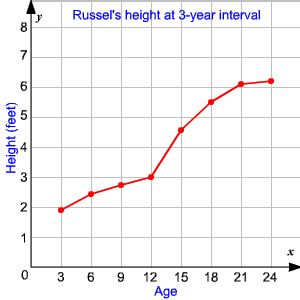# Line Graphs

A line graph displays data using line segments. Line graphs are mostly used to display data that changes over a period of time.

Example:

The table shows Russel's height at $3$ -year interval. Make a line graph to display the data.

 Age $3$ $6$ $9$ $12$ $15$ $18$ $21$ $24$ Height (feet) $1.9$ $2.4$ $2.7$ $3$ $4.6$ $5.5$ $6.1$ $6.2$

To draw a line graph, first draw a horizontal and a vertical axis. Age should be plotted on the horizontal axis because it is independent. Height should be plotted on the vertical axis.

Then look for the given data and plot a point for each pair of values. Draw a line connecting each pair of consecutive points. Then title the graph and label the horizontal and vertical axis.